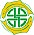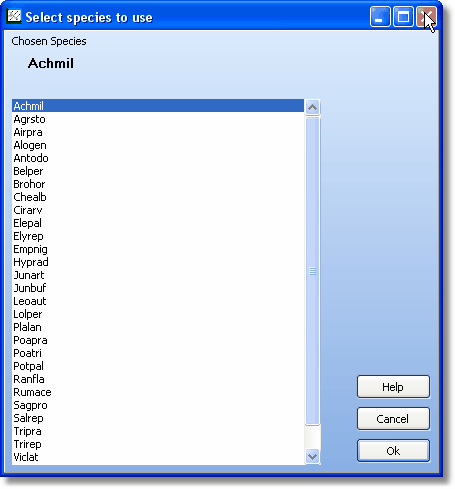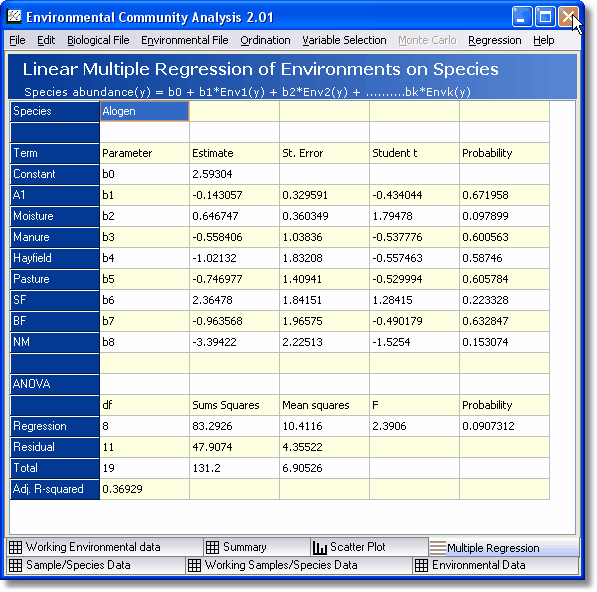Multiple Regression
 Select the species against which to run the regression in the lookup box.The multiple regression results tab is displayed once the Multiple Regression method has been run by selecting Regression|Multiple Regression from the drop-down menus at the top of the main form. This window presents the results of the multiple linear regression analysis of the environmental parameters on the abundance of the selected species. The environmental parameters are the independent variables and the species abundance is the dependent variable.The fitted equation is of the form:   Species abundance (y) = b0 + b1*Environment 1 + b2*Environment 2 +............ bk*Environment k,   where b0, b1,b2.....bk are the fitted parameters.   The upper part of the output grid gives the estimated parameters, their standard error, the Student t value and its estimated probability. To be significant at the 5% level a parameter should have a probability < 0.05.   The lower part of the grid gives the Analysis of variance table, the F value and its probability. The regression explains a significant part of the variation in species number at the 5% level if the probability value is < 0.05.   Pearson correlations between any two variables (biological or environmental) can be obtained from the scatter plot tab - see Scatter Plot.